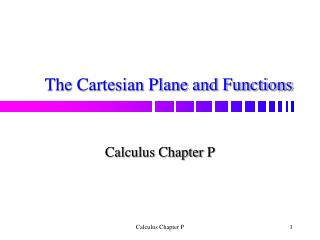DownloadDownload PresentationThe Cartesian Plane and Functions

# The Cartesian Plane and Functions

Télécharger la présentation## The Cartesian Plane and Functions

- - - - - - - - - - - - - - - - - - - - - - - - - - - E N D - - - - - - - - - - - - - - - - - - - - - - - - - - -
##### Presentation Transcript

1. The Cartesian Plane and Functions Calculus Chapter P Calculus Chapter P

2. Real line • Number line • X-axis Calculus Chapter P

3. Coordinate • The real number corresponding to a point on the real line Calculus Chapter P

4. Origin • zero Calculus Chapter P

5. Positive direction • To the right • Shown by arrowhead • Direction of increasing values of x Calculus Chapter P

6. Nonnegative • Positive or zero Calculus Chapter P

7. Nonpositive • Negative or zero Calculus Chapter P

8. One-to-one correspondence • Type of relationship • Example: each point on the real line corresponds to one and only one real number, and each real number corresponds to one and only one point on the real line Calculus Chapter P

9. Rational numbers • Can be expressed as the ratio of two integers • Can be represented by either a terminating decimal or a repeating decimal Calculus Chapter P

10. Irrational numbers • Not rational • Cannot be represented as terminating or repeating decimals Calculus Chapter P

11. Order and inequalities • Real numbers can be ordered • If a and b are real numbers, then a is less than b if b – a is positive • Shown with inequality a < b Calculus Chapter P

12. Properties of inequalities • Page 2 Calculus Chapter P

13. Set • A collection of elements Calculus Chapter P

14. Subset • Part of a set Calculus Chapter P

15. Set notation • The set of all x such that a certain condition is true • {x : condition on x} • Negative numbers : {x : x < 0} Calculus Chapter P

16. Union of sets A and B • The set of elements that are members of A or B or both Calculus Chapter P

17. Intersections of sets A and B • The set of elements that are members of A and B Calculus Chapter P

18. Disjoint sets • Have no elements in common Calculus Chapter P

19. Open interval • Endpoints are not included Calculus Chapter P

20. Closed Interval • Endpoints are included Calculus Chapter P

21. Types of intervals • See page 3 Calculus Chapter P

22. 1. Example • Exercise 16 Calculus Chapter P

23. 2. Example • Solve and sketch the solution on the real line. Calculus Chapter P

24. 3. You try • Solve and sketch the solution on the real line. Calculus Chapter P

25. 4. Example • Solve Calculus Chapter P

26. 5. You try • Solve Calculus Chapter P

27. Polynomial inequalities • Remember that a polynomial can change signs only at its real zeros • Find zeros, then use them to divide real line into test intervals • Test one value in each interval to determine if it makes the inequality true or not Calculus Chapter P

28. 6. Example Calculus Chapter P

29. 7. You try Calculus Chapter P

30. Absolute value • See page 6 Calculus Chapter P

31. Absolute value inequalities • Rewrite as a double inequality Calculus Chapter P

32. 8. Example Calculus Chapter P

33. 9. You try Calculus Chapter P

34. Distance between a and b Calculus Chapter P

35. Directed distances • From a to b is b – a • From b to a is a – b Calculus Chapter P

36. 10. You try • Find the distance between –5 and 2 • Find the directed distance from –5 to 2 • Find the directed distance from 2 to –5 Calculus Chapter P

37. Midpoint of an interval Calculus Chapter P

38. To prove • Show that the midpoint is equidistant from a and b Calculus Chapter P

39. The Cartesian Plane Calculus P.2 Calculus Chapter P

40. Cartesian Plane • Rectangular coordinate system • Named after René Descartes • Ordered pair: (x, y) • Horizontal x-axis • Vertical y-axis • Origin: where axes intersect Calculus Chapter P

41. Quadrants II I IV III Calculus Chapter P

42. Distance formula • Pythagorean theorem d Calculus Chapter P

43. 1. You try • Find the distance between (-3, 2) and (3, -2) Calculus Chapter P

44. Midpoint formula • To find the midpoint of the line segment joining two points, average the x-coordinates and average the y-coordinates. • Midpoint has coordinates Calculus Chapter P

45. Circle • The set of all points in a plane that are equidistant from a fixed point. • Center: the fixed point • Radius: distance from fixed point to point on circle Calculus Chapter P

46. Equation for a circle Standard form Calculus Chapter P

47. Circles • If the origin is at (0, 0), then Calculus Chapter P

48. Unit circle • Center at origin and radius of 1 Calculus Chapter P

49. General Form • Obtained from standard form by squaring and simplifying. • To convert from general form to standard form, you must complete the square. • If you get a radius of 0, then it is a single point. • If you get a negative radius, then the graph does not exist. Calculus Chapter P

50. Completing the square • Get coefficients of x2 and y2 to be 1. • Get variable terms on one side of the equation and constant terms on the other. • Add the square of half the coefficient of x and the square of half the coefficient of y to both sides. • Factor and simplify. Calculus Chapter P